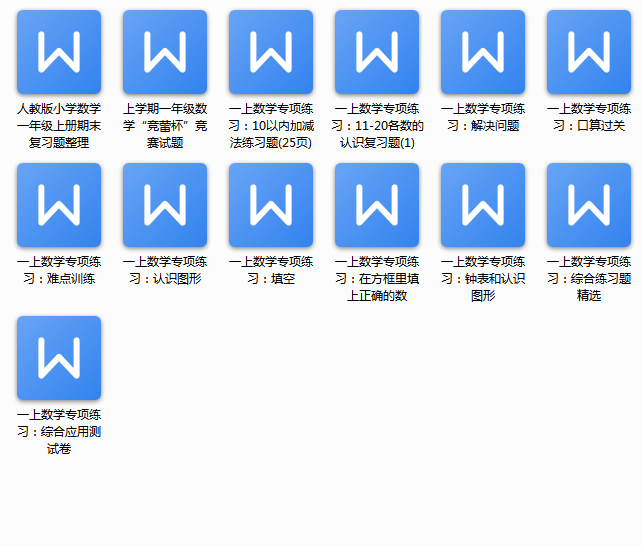2+2=       4-1=      3-2=      0+1=       5-1=

3+2=　　　 4+1=      1+1=      4-0=       5-2=

1.数一数，圈一圈。（8分，每空2分）